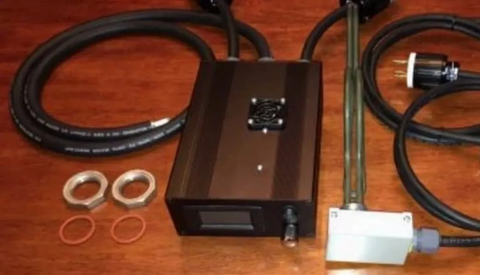• Oct 28, 2020
• by MoonshinerWelcome back, So we're gonna talk about a topic that's going to be helpful to everybody. We did a discussion on evaluating the circuit to make sure that you've got the proper amperage, you're using the proper amount of amperage or you're not overloading that circuit, just to make sure you're safe and you're not going to blow a circuit. It's going to be efficient, effective, and you're going to be successful.

Now, we're going to do something that's really, neat, because there's a shortcut to most of this that you can use when you're at the store. Now it all works out the same but with this, you'll understand how to do an entire circuit with everything else that you've got plugged into it. You can figure it all out. But with this, we're gonna do just isolating these.

Now two things are a couple of things you might want to know about heater elements. Heater elements come in a wide variety. But the primary two differences are, you're gonna have a high density or you're gonna have a low density.

What's the difference? A high density has less cubic inches surface area. So that means that each cubic inch has to burn a little bit hotter, and a low density has more surface area, but cubic inches of its surface area. So that means each cubic inch can burn a little bit cooler. But primarily, the difference between the two is absolutely nothing. They both do exactly the same thing. They heat exactly in the same manner. And sometimes, the low densities are probably more advantageous for water heaters when you've got like really hard water or something like that. They don't burn out as quickly as a high density.

And now you remember this hot mess that we went through a new VWV a r or p, e, IR. And that's just the things that we know about electricity, the four basic values we know about electricity. This is what you may be faced with when you come across all those heater elements that you're trying to figure out and you got to try to design for your still. And so you'll have 120 volt category, and they come in 1000 1500 and 2000 watt. You've got the 240 volts that remember you can wire these at 120 volts, but what happens is is a 3000-watt times point two five because you lose 75% of the wattage is really a 750-watt element now and there's a good reason for that that you need to know that the 3500 watt is 875 watts and you'll see how these break down. And then your third category is The 240 volt, that's 3030 540 550 500 watts. And they come in many different variations.

So you're standing there in front of the kit, and you've got this little calculator when you're like, p divided by E. And remember we change those, we change those letters, so we made them wattage divided by voltage equals amps.

Now, what combination and I want to use two elements. Let's say I want to use one element. Well, can I use 120 volts or 1500 watt element. Remember, we said the wattage divided by the voltage 1500 divided by 120 equals 12.5 amps. So this one would be a 12.5 amp on that 20 amp circuit, you know you're safe.

For instance, you're going to do well let's do a 240. Because the 240 works the same way. We'll do the 3500 watt 3500 divided by 240 equals 14.58 amps on that 20 amp circuit. We want to do two elements. I'm going to do two elements, I'm going to do 215 hundred watt elements on my 120 volt system. Because of the relationship, remember, we talked the other one was there was an inverse relationship with resistance.

There's a direct relationship with power in wattage. So we take 1500 we've got two of them. That's 3000 Got 3000 watts now and we divide that by 120. That equals 25 amps. So we're a little bit high. So now we got to figure out what combination Can I use? A 1500 and a 3500 wired at 120, which is now 875. So 1500 plus 875 equals and then divided by 120. That's 19.7 amps.

I am under my 20 amp threshold, but I'm really close. So with this combination of these two elements right here, if I've got a two-element system on 120-volt system, theoretically, that's going to work well but It's like 19.7 amps at max draw. I'd like to get it a little bit lower. So right Now amid 19.7 amps, what do I do? The 1500 watt and the 3000 watt wide at 120, which is now 750. I love this stuff 1500 plus 750 equals divided by 120. Now I'm at 18.75 amps. So I've dropped an amp. And I feel much safer with that, because I've got two elements now on that 120 volt circuit.

Now this will work in any combination. Let's do a 3000 and a 3500 or 240 volts system and we'll say this one's on a 30 amp breaker. This one's on a 30 amp breaker that would be 6500 watts and we divide that by 240 volts We get 27.08 amps. So if we had these two wired together under 240 volts, it would be 27.08 amps. And overall a 30 amp circuit or absolutely safe.

So think about that when you're standing in that aisle and you're looking at all of those combinations and you just want it. What am I going to do or you can do the math quickly at home before you even get there.